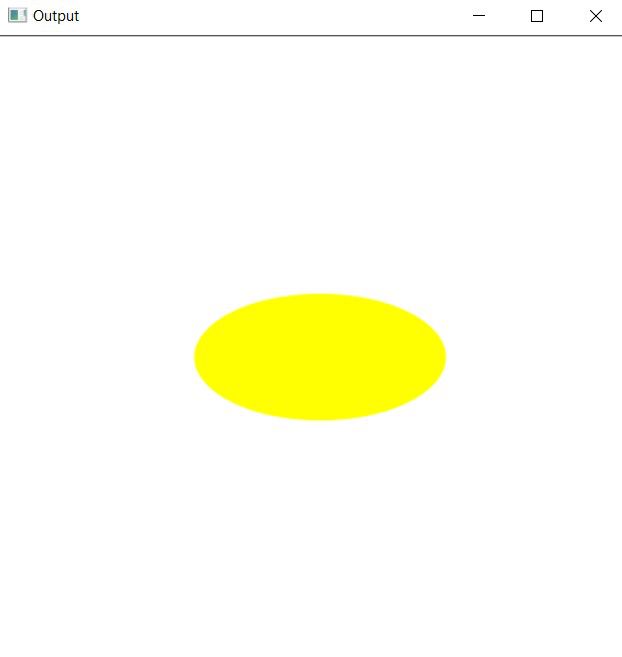# Draw an ellipse using OpenCV in C++

• Difficulty Level : Hard
• Last Updated : 22 Feb, 2021

In this article, the task is to draw an ellipse using OpenCV in C++. The ellipse() function from OpenCV C++ library will be used.

Syntax:

ellipse(image, centerCoordinates, axesLength, angle, startAngle, endAngle, color, thickness, lineType, shift)

Parameters:

• image: It is the image on which ellipse is to be drawn.
• centerCoordinates: Coordinates of the center of the ellipse. (Tuple of two coordinates (X-coordinate, Y-coordinate))
• axesLength:Tuple containing the major and minor axis of the ellipse (major axis length, minor axis length).
• angle: Ellipse rotation angle in degrees.
• startAngle: Starting angle of the elliptic arc(degrees).
• endAngle: Ending angle of the elliptic arc(degrees).
• color: It is the color of the borderline of the ellipse to be drawn. A tuple representing 3 colors (B, G, R) i.e., (Blue, Green, Red).
• thickness: It is the thickness of the ellipse borderline in px. The thickness of -1 px will fill the ellipse shape by the specified color.
• lineType: Type of the line. There are 3 types of line:
• LINE_4: Line was drawn using 4 connected Bresenham algorithm.
• LINE_8: Line was drawn using 8 connected Bresenham algorithm.
• LINE_AA: It draws Anti-aliased lines formed by using a Gaussian filter.
• shift: Number of fractional bits in the point coordinates.

Return Value: It returns an image.

Program 1:

Below is the C++ program demonstrating how to draw an ellipse over a self-formed background image:

## C++

 `// C++ program to demonstrating ellipse ` `// over a self-formed background image ` `#include ` `#include ` ` `  `// Drawing shapes ` `#include ` ` `  `#include ` `using` `namespace` `cv; ` `using` `namespace` `std; ` ` `  `// Driver Code ` `int` `main(``int` `argc, ``char``** argv) ` `{ ` `    ``// Creating a blank image with ` `    ``// white background ` `    ``Mat image(500, 500, CV_8UC3, ` `              ``Scalar(255, 255, 255)); ` ` `  `    ``// Check if the image is created ` `    ``// successfully or not ` `    ``if` `(!image.data) { ` `        ``std::cout << ``"Could not open or "` `                  ``<< ``"find the image\n"``; ` ` `  `        ``return` `0; ` `    ``} ` ` `  `    ``// Drawing the ellipse ` `    ``ellipse(image, Point(256, 256), ` `            ``Size(100, 50), 0, 0, ` `            ``360, Scalar(0, 255, 255), ` `            ``-1, LINE_AA); ` ` `  `    ``// Showing image inside a window ` `    ``imshow(``"Output"``, image); ` `    ``waitKey(0); ` ` `  `    ``return` `0; ` `} `

Output:Explanation: In the above program, an ellipse is drawn at an angle of 0 degrees i.e., horizontal ellipse.

Program 2:

Below is the C++ program illustrate rectangle over a loaded image with an ellipse around the GFG logo:

## C++

 `// C++ program to demonstrate rectangle ` `// over a loaded image with an ellipse ` `// around the GFG logo ` `#include ` `#include ` ` `  `// Drawing shapes ` `#include ` ` `  `#include ` `using` `namespace` `cv; ` `using` `namespace` `std; ` ` `  `// Driver Code ` `int` `main(``int` `argc, ``char``** argv) ` `{ ` `    ``// Reading the Image ` `    ``Mat image = imread(``"C:/Users/harsh/Downloads/geeks.png"``, ` `                       ``IMREAD_COLOR); ` ` `  `    ``// Check if the image is created ` `    ``// successfully or not ` `    ``if` `(!image.data) { ` `        ``std::cout << ``"Could not open or "` `                  ``<< ``"find the image\n"``; ` `        ``return` `0; ` `    ``} ` ` `  `    ``// Drawing the ellipse ` `    ``ellipse(image, Point(115, 110), ` `            ``Size(105, 55), 0, 0, ` `            ``360, Scalar(0, 255, 255), ` `            ``1, LINE_AA); ` ` `  `    ``// Show our image inside a window ` `    ``imshow(``"Output"``, image); ` `    ``waitKey(0); ` ` `  `    ``return` `0; ` `} `

Output:My Personal Notes arrow_drop_up
Recommended Articles
Page :